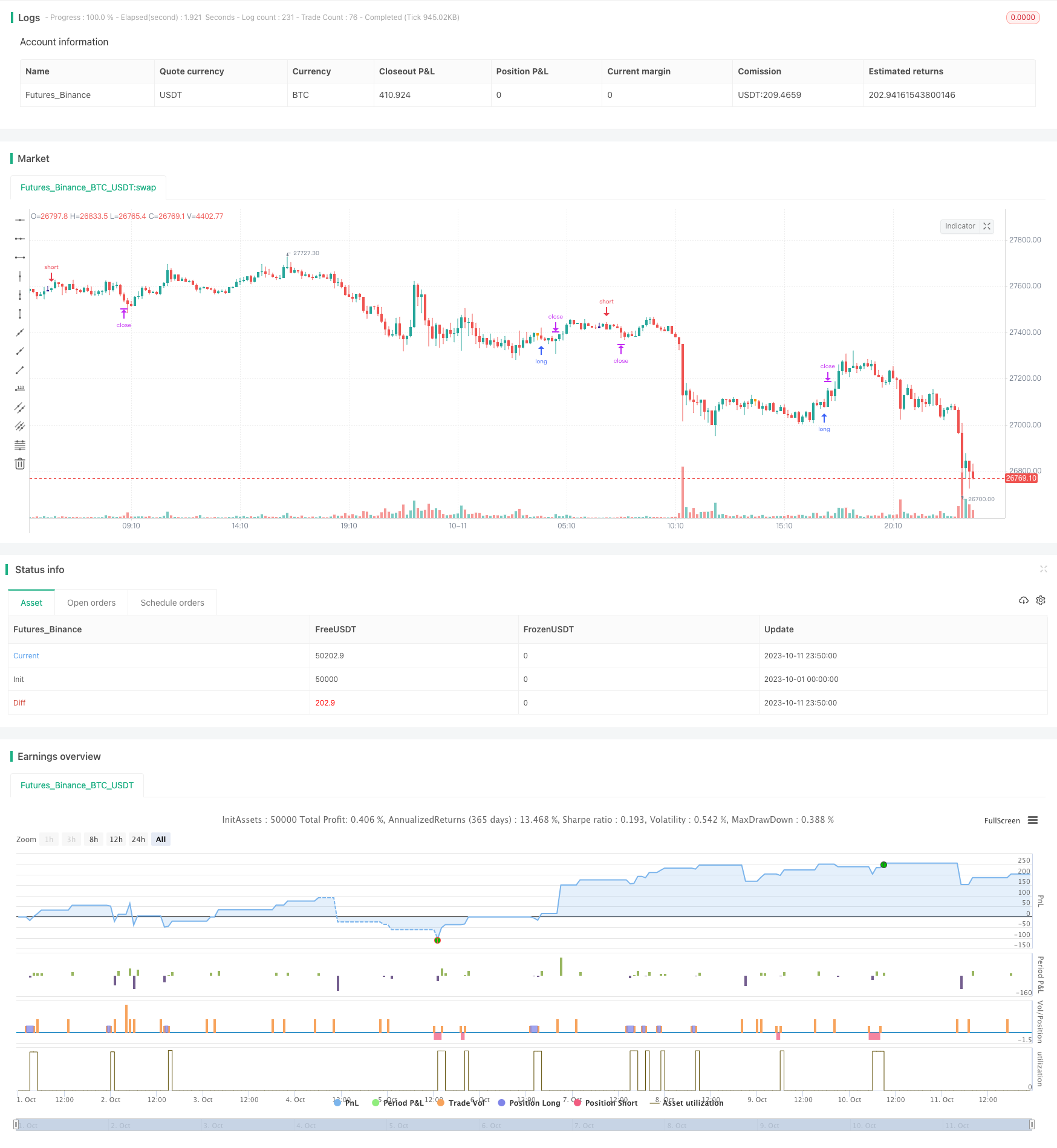Author: ChaoZhang, Date: 2023-11-03 16:03:59
Tags:## Overview

The shadow trading strategy identifies K-line with long lower or upper shadows to determine potential market reversal opportunities. It goes long when a long lower shadow is identified and goes short when a long upper shadow is identified. The strategy mainly utilizes the general principle of shadow reversal for trading.

## Strategy Logic

The core logic of the shadow trading strategy is to identify long upper and lower shadows in K-lines. The strategy calculates the size of the K-line body `corpo` and the size of the shadows `pinnaL` and `pinnaS`. When the size of the shadow is larger than the body size by a certain multiplier, it considers there may be reversal opportunities. Specifically, the strategy includes the following steps:

1. Calculate K-line body size `corpo`, which is the absolute value of the difference between open and close price.
2. Calculate upper shadow `pinnaL`, which is the absolute value of the difference between highest price and close price.
3. Calculate lower shadow `pinnaS`, which is the absolute value of the difference between lowest price and close price.
4. Check if upper shadow is larger than body size by a multiplier, through `pinnaL > (corpo*size)`, where `size` is an adjustable parameter.
5. Check if lower shadow is larger than body size by a multiplier, through `pinnaS > (corpo*size)`.
6. If above conditions are met, go short (long upper shadow) or long (long lower shadow) at the close of the K-line with shadow.

In addition, the strategy also checks if the K-line range `dim` is greater than the minimum value `min` to filter out trivial K-lines with negligible range. Stop loss and take profit are used for exit.

• Utilizes the general principle of shadow reversal, which is a relatively reliable trading signal
• Simple and clear strategy logic, intuitive parameter settings, easy to grasp
• Flexible risk control by adjusting parameters to change entry frequency
• Can be further optimized by combining trend, support/resistance etc.

## Risks and Solutions

• Probability of failure in shadow reversal exists, can lower risk by adjusting parameters
• Needs combination with trend judgment to avoid counter trend trades
• Parameters need optimization for different products, may vary across products
• Can combine other indicators to filter entries, lower win rate for higher profitability

## Optimization Directions

• Optimize parameters by product to improve stability
• Add trend judgment with moving averages etc. to avoid counter trend
• Add checks on breaking recent high/low points to improve efficacy
• Optimize stop loss and take profit to maximize profit while minimizing loss
• Optimize position sizing, can vary across different products

## Conclusion

```/*backtest
start: 2023-10-01 00:00:00
end: 2023-10-11 23:59:59
period: 10m
basePeriod: 1m
exchanges: [{"eid":"Futures_Binance","currency":"BTC_USDT"}]
*/

//@version=2

size = input(1,type=float)
pinnaL = abs(high - close)
pinnaS = abs(low-close)
scarto = input(title="Tail Tollerance", type=float, defval=0.0018)
corpo = abs(close - open)
dim = abs(high-low)
min = input(0.001)
shortE = (open + dim)

longE = (open - dim)
barcolor(dim > min and (close > open) and (pinnaL > (corpo*size)) and (open-low<scarto) ? navy : na)

longcond = (dim > min) and (close > open) and (pinnaL > (corpo*size)) and (open-low<scarto)
minimo=low+scarto
massimo=high+scarto
barcolor( dim > min and(close < open) and (pinnaS > (corpo*size)) and (high-open<scarto) ? orange: na)
shortcond = (dim > min) and(close < open) and (pinnaS > (corpo*size)) and (high-open<scarto)
//plot(shortE)
//plot(longE)
//plot(open)
ss= shortcond ? close : na
ll=longcond ? close : na
offset= input(0.00000)

DayClose = 2

longCondition = (close > open) and (pinnaL > (corpo*size)) and (open-low<scarto)

crossFlag = longcond ? 1 : 0
monthBegin = input(1,maxval = 12)
yearBegin = input(2013, maxval= 2015, minval=2000)

if(month(time)>monthBegin and year(time) >yearBegin)
if (longcond)
strategy.entry("short", strategy.short, stop = low - offset)
//strategy.close("short", when = closup)
shortCondition = (close < open) and (pinnaS > (corpo*size)) and (high-open<scarto)
if(month(time)>monthBegin and year(time) >yearBegin)
if (shortcond)
strategy.entry("long", strategy.long, stop = high + offset)
//strategy.close("long", when = closup)

Target =  input(20)
Stop = input(70) //- 2
Trailing = input(0)
CQ = 100

TPP = (Target > 0) ? Target*10: na
SLP = (Stop > 0) ? Stop*10 : na
TSP = (Trailing > 0) ? Trailing : na

strategy.exit("Close Long", "long", qty_percent=CQ, profit=TPP, loss=SLP, trail_points=TSP)
strategy.exit("Close Short", "short", qty_percent=CQ, profit=TPP, loss=SLP, trail_points=TSP)
```

More